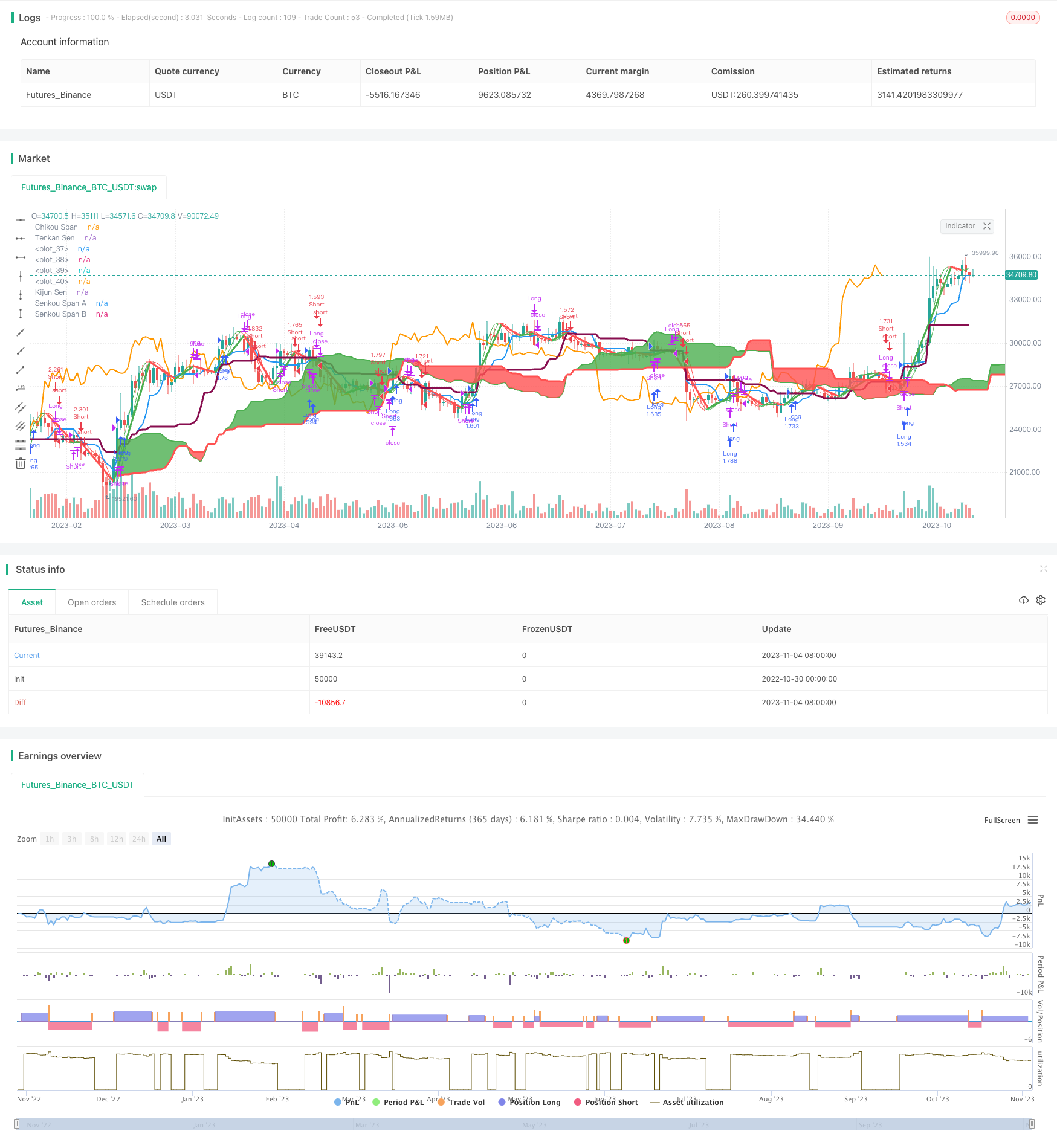# Random Oscillation Strategy

Author: ChaoZhang, Date: 2023-11-06 09:30:27
Tags:## Overview

The Random Oscillation Strategy integrates multiple technical indicators, including Ichimoku Kinko Hyo, MACD and Hull Moving Average, to form a systematic trading decision system. It aims to capture trend reversal points and potential opportunities during oscillating markets.

## Strategy Logic

Firstly, the Tenkan-sen and Kijun-sen of Ichimoku Kinko Hyo are adopted. Tenkan-Sen is calculated as the mean of the highest high and lowest low over the past 9 periods. Kijun-Sen is the mean of the highest high and lowest low over the past 24 periods. The crossovers of price and Kijun-sen act as trading signals.

Secondly, the MACD indicator is incorporated as an important trend-following momentum indicator. It shows the relationship between two EMAs of prices. Crossovers of MACD and its signal line generate trading signals.

Thirdly, the Hull Moving Average is introduced to improve the lagging issue of moving averages and increase sensitivity of catching price reversals. It is calculated using WMAs of half, full and square root periods. Crossovers between fast and slow Hull MAs also act as auxiliary signals.

Lastly, the strategy combines all indicators above to form a robust trading system. Actual entries and exits only occur when multiple indicators give unanimous signals.

• Diversification via multiple indicators reduces single point failure.

• Integration provides stronger decision power through holistic model.

• Decreased false signals as every signal is verified by others.

• Improved efficiency by only acting on high-conviction signals.

• Customizable parameters to adapt the strategy to changing markets.

• Reduced lag and quicker response from Hull Moving Average.

## Risks

• Higher risk in ranging, choppy markets with increased false signals.

• Ineffective if indicator parameters are not properly optimized.

• Potentially misses trending moves by focusing on reversals.

• Hull MA is relatively new and unproven in the long run.

• Infrequent trading could miss some opportunities.

## Enhancement

• Adding more indicators like Bollinger Bands could further optimize the system.

• Parameter tuning to find the optimal combination for different assets and timeframes.

• Introduce dynamic stops to control single trade loss.

• Incorporate trend filters to avoid missing trend rides.

• Optimize position sizing by adjusting frequency and size based on market conditions.

## Conclusion

The Random Oscillation Strategy combines multiple technical analysis techniques to capture opportunities in range-bound markets. It offers the advantages of indicator integration, reduced false signals and improved efficiency. But it also carries inherent risks that require further optimization and adaption. Overall, it represents a robust, practical approach for trading oscillating markets.

```/*backtest
start: 2022-10-30 00:00:00
end: 2023-11-05 00:00:00
period: 1d
basePeriod: 1h
exchanges: [{"eid":"Futures_Binance","currency":"BTC_USDT"}]
*/

//@version=2
strategy("Ichimoku Kinko Hyo + HULL-MA_X + MacD", shorttitle="@m", overlay=true, default_qty_type=strategy.percent_of_equity, max_bars_back=1000, default_qty_value=100, calc_on_order_fills= true, calc_on_every_tick=true, pyramiding=0)

keh=input(title="Double HullMA",defval=12, minval=1)

n2ma=2*wma(close,round(keh/2))
nma=wma(close,keh)
diff=n2ma-nma
sqn=round(sqrt(keh))
n2ma1=2*wma(close,round(keh/2))
nma1=wma(close,keh)
diff1=n2ma1-nma1
sqn1=round(sqrt(keh))
n1=wma(diff,sqn)
n2=wma(diff1,sqn)
b=n1>n2?lime:red
c=n1>n2?green:red
d=n1>n2?red:green

TenkanSenPeriods = input(9, minval=1, title="Tenkan Sen Periods")
KijunSenPeriods = input(24, minval=1, title="Kijun Sen Periods")
SenkouSpanBPeriods = input(51, minval=1, title="Senkou Span B Periods")
displacement = input(24, minval=1, title="Displacement")
donchian(len) => avg(lowest(len), highest(len))
TenkanSen = donchian(TenkanSenPeriods)
KijunSen = donchian(KijunSenPeriods)
SenkouSpanA = avg(TenkanSen, KijunSen)
SenkouSpanB = donchian(SenkouSpanBPeriods)
LS=close, offset = -displacement

MACD_Length = input(9)
MACD_fastLength = input(12)
MACD_slowLength = input(24)
MACD = ema(close, MACD_fastLength) - ema(close, MACD_slowLength)
aMACD = ema(MACD, MACD_Length)

a1=plot(n1,color=c)
a2=plot(n2,color=c)
plot(cross(n1, n2) ? n1 : na, style = circles, color=b, linewidth = 4)
plot(cross(n1, n2) ? n1 : na, style = line, color=d, linewidth = 3)
plot(TenkanSen, color=blue, title="Tenkan Sen", linewidth = 2)
plot(KijunSen, color=maroon, title="Kijun Sen", linewidth = 3)
plot(close, offset = -displacement, color=orange, title="Chikou Span", linewidth = 2)
p1=plot (SenkouSpanA, offset = displacement, color=green,  title="Senkou Span A", linewidth = 2)
p2=plot (SenkouSpanB, offset = displacement, color=red,  title="Senkou Span B", linewidth = 3)
fill(p1, p2, color = SenkouSpanA > SenkouSpanB ? green : red)

closelong = n1<n2 and close<n2 and (MACD<aMACD or TenkanSen<KijunSen or close<KijunSen)
if (closelong)
strategy.close("Long")

closeshort = n1>n2 and close>n2 and (MACD>aMACD or TenkanSen>KijunSen or close>KijunSen)
if (closeshort)
strategy.close("Short")

longCondition = n1>n2 and close>n2 and MACD>aMACD and (TenkanSen>KijunSen or close>KijunSen)
if (longCondition)
strategy.entry("Long",strategy.long)

shortCondition = n1<n2 and close<n2 and MACD<aMACD and (TenkanSen<KijunSen or close<KijunSen)
if (shortCondition)
strategy.entry("Short",strategy.short)
```

More